Courses

# Second Law Of Termodynamics – MSQ

## 10 Questions MCQ Test Topic wise Tests for IIT JAM Physics | Second Law Of Termodynamics – MSQ

Description
This mock test of Second Law Of Termodynamics – MSQ for Physics helps you for every Physics entrance exam. This contains 10 Multiple Choice Questions for Physics Second Law Of Termodynamics – MSQ (mcq) to study with solutions a complete question bank. The solved questions answers in this Second Law Of Termodynamics – MSQ quiz give you a good mix of easy questions and tough questions. Physics students definitely take this Second Law Of Termodynamics – MSQ exercise for a better result in the exam. You can find other Second Law Of Termodynamics – MSQ extra questions, long questions & short questions for Physics on EduRev as well by searching above.
*Multiple options can be correct
QUESTION: 1

### Which of the following is/are correct statement? Select one or more:

Solution:

All reversible engines have same efficiency only if they operate between same temperature and is given by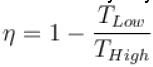The correct answer is: All the reversible engines operating between same temperatures have same efficiency

*Multiple options can be correct
QUESTION: 2

### Entropy does not remains constant in which of the following processes? Select one or more:

Solution:

In adiabatic process, dQ = 0
⇒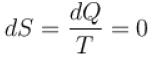⇒ S remains constant.
The correct answers are: Isothermal process, Cyclic process, Isobaric process

*Multiple options can be correct
QUESTION: 3

### The main cause of irreversibility is/are. Select one or more:

Solution:

All the 3 options (a, b & c) results in the loss of heat and thus, result in irreversibility.
The correct answers are: mechanical and fluid friction, unrestricted expansion, heat transfer with a finite temperature difference

*Multiple options can be correct
QUESTION: 4

Which of the following is a property of entropy?
Select one or more:

Solution:

From second law of thermodynamics, (a) and (b) are true.
In adiabatic process, dQ = 0
⇒ dS = 0, hence (c) is also true.
The correct answers are: Entropy increases during an irreversible operation   , Net change in entropy in a reversible cycle is zero, Change in entropy during an adiabatic operation is zero

*Multiple options can be correct
QUESTION: 5

A Carnot cycle operates on a working substance between two reservoirs at temperature T1 and T2, where T1 >T2. During each cycle, an amount of heat Q1 is extracted from the reservoir at T1 and an amount Q2 is delivered to the reservoir at T2. Which of the following statements are correct?
Select one or more:

Solution:

Here, the only false statement is efficiency is equal to 1
∴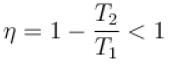The correct answers are: work done in one cycle is Q1 – Q2, entropy of the hotter reservoir decreases, entropy for the universe (consisting of the working substance and the two reservoirs) increases

*Multiple options can be correct
QUESTION: 6

The condition for the reversibility of a cycle is.
Select one or more:

Solution:

All the four options given above are necessary for reversibility of a cycle

The correct answers are: The pressure and temperature of working substance must not differ, appreciably from those of the surroundings at any stage in the process, All the processes taking place in the cycle of operation, must be extremely slow, The working parts of the engine must be friction free, There should be no loss of energy during the cycle of operation

*Multiple options can be correct
QUESTION: 7

Which of the following statements are correct?
Select one or more:

Solution:

(a, b, c) are all different definitions associated with the entropy and are thus all correct
The correct answers are: The increase in entropy is obtained from a given quantity of heat at a low temperature, The change in entropy may be regarded as a measure of the rate of the availability of heat for transformation into work., The entropy represents the maximum amount of work obtainable per degree drop in temperature

*Multiple options can be correct
QUESTION: 8

For any process, the second law of thermodynamics requires that the change of entropy of the universe be.
Select one or more:

Solution:

In reversible processes, change in entropy is zero but in irreversible process, change in entropy is positive. So, for any process, the change of entropy of the universe will be positive or zero.
The correct answers are: positive, zero

*Multiple options can be correct
QUESTION: 9

Out of three Carnot engines, operating between reservoir temperature of (a) 400K and 500K (b) 600K and 800K (c) 800K and 1000K, which has/have the lowest thermal efficiency?
Select one or more:

Solution: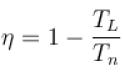for (a),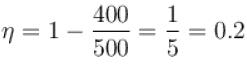for (b),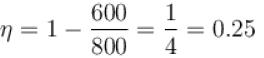for (c),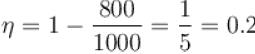a, c

*Multiple options can be correct
QUESTION: 10

According to Carnot’s theorem.
Select one or more:

Solution:

Efficiency of Carnot engine doesn’t depend on working substance and its efficiency is always less than unity.
The correct answers are: Efficiency of a Carnot engine solely depends on hot and cold temperature reservoirs, Carnot engine has the maximum possible efficiency